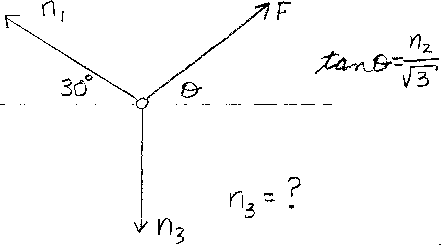Problem A4: In the figure above, three forces act on a ring. The net force on the ring is zero. One force is at an angle of 30 degrees with a magnitude of n1 Newtons. A second force F acts at an angle &theta . The tangent of the angle is tan &theta = n2 / √ 3 . If the third force is n3 Newtons, what is n3?n1 = n2 = Input n3:

If you are currently in my class, you can record your grade by entering your name and student ID number (without the leading zeros) below and clicking on "record grade".
 First Name = Last Name = ID = Problem: